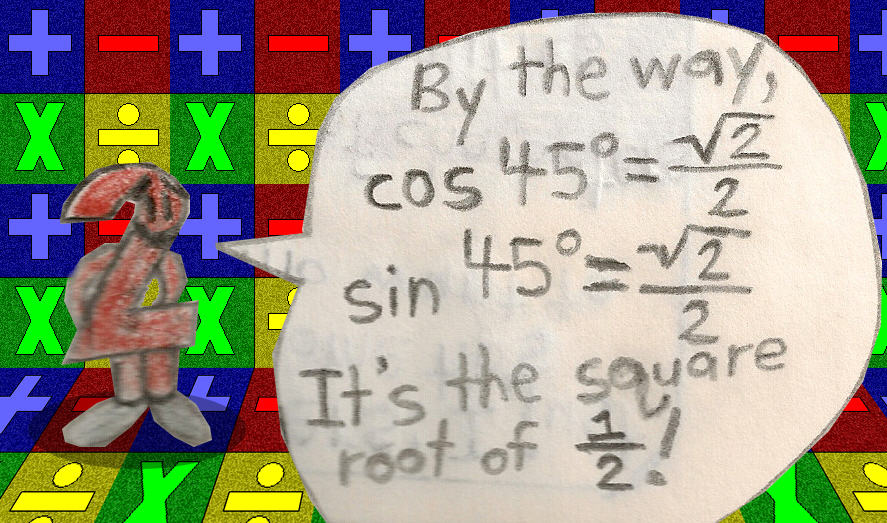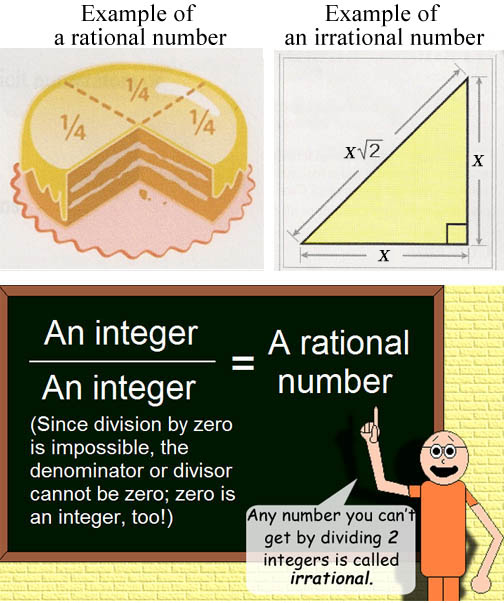# Trigonometry Tricks

## Examples:

cos 75° = .259...

cos 15° = .259...

cos 45° = .707...

sin 45° = .707...

(Notice that the sine & cosine of 45 degrees are exactly the same!)I almost forgot that most of the time, sines, cosines, tangents and such are irrational numbers; this explains the triple periods after the numbers in the examples.

## Something to remember about real numbers: